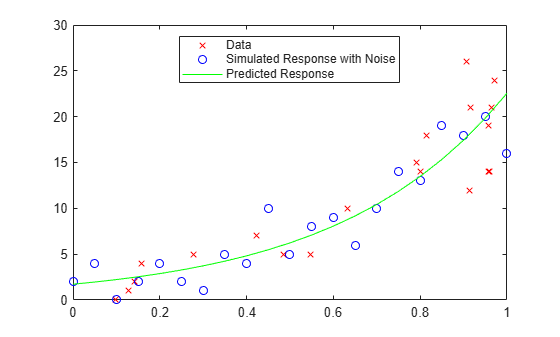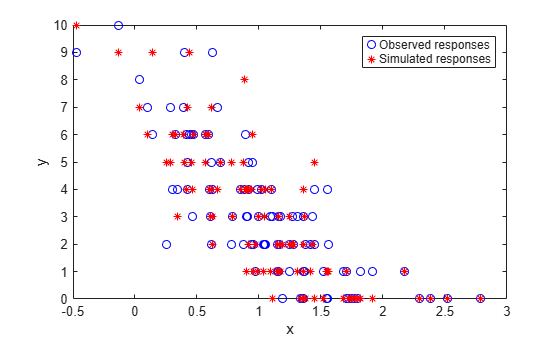# random

Simulate responses with random noise for generalized linear regression model

## Syntax

``ysim = random(mdl,Xnew)``
``ysim = random(mdl,Xnew,Name,Value)``

## Description

example

````ysim = random(mdl,Xnew)` simulates responses to the predictor data in `Xnew` using the generalized linear regression model `mdl`, adding random noise.```

example

````ysim = random(mdl,Xnew,Name,Value)` specifies additional options using one or more name-value pair arguments. For example, you can specify the number of trials for binomial distribution or the offset value used for fitting.```

## Examples

collapse all

Create a generalized linear regression model, and simulate its response with random noise to new data.

Generate sample data using Poisson random numbers with one underlying predictor `X`.

```rng('default') % For reproducibility X = rand(20,1); mu = exp(1 + 2*X); y = poissrnd(mu);```

Create a generalized linear regression model of Poisson data.

`mdl = fitglm(X,y,'y ~ x1','Distribution','poisson');`

Create data points for prediction.

`Xnew = (0:.05:1)';`

Simulate responses with random noise at the data points.

`ysim = random(mdl,Xnew);`

Plot the simulated values and the original values.

```plot(X,y,'rx',Xnew,ysim,'bo',Xnew,feval(mdl,Xnew),'g-') legend('Data','Simulated Response with Noise','Predicted Response', ... 'Location','best')```Fit a generalized linear regression model, and then save the model by using `saveLearnerForCoder`. Define an entry-point function that loads the model by using `loadLearnerForCoder` and calls the `predict` function of the fitted model. Then use `codegen` (MATLAB Coder) to generate C/C++ code. Note that generating C/C++ code requires MATLAB® Coder™.

This example briefly explains the code generation workflow for the prediction of linear regression models at the command line. For more details, see Code Generation for Prediction of Machine Learning Model at Command Line. You can also generate code using the MATLAB Coder app. For details, see Code Generation for Prediction of Machine Learning Model Using MATLAB Coder App.

Train Model

Generate sample data of the predictor `x` and response `y` with the following distributions:

• $x\sim N\left(1,0.{5}^{2}\right)$.

• ${\beta }_{0}=1$ and $\beta =-2$.

• $y\sim Binomial\left(10,\frac{exp\left(1+x\beta \right)}{1+exp\left(1+x\beta \right)}\right)$.

```rng('default') % For reproducibility x = 1 + randn(100,1)*0.5; beta = -2; p = exp(1 + x*beta)./(1 + exp(1 + x*beta)); % Inverse logit n = 10; y = binornd(n,p,100,1);```

Create a generalized linear regression model of binomial data. Specify a binomial sample size of 10.

`mdl = fitglm(x,y,'y ~ x1','Distribution','Binomial','BinomialSize',n);`

Save Model

Save the fitted generalized linear regression model to the file `GLMMdl.mat` by using `saveLearnerForCoder`.

`saveLearnerForCoder(mdl,'GLMMdl');`

Define Entry-Point Function

In your current folder, define an entry-point function named `myrandomGLM.m` that does the following:

• Accept new predictor input and valid name-value pair arguments.

• Load the fitted generalized linear regression model in `GLMMdl.mat` by using `loadLearnerForCoder`.

• Simulate responses from the loaded GLM model.

```function y = myrandomGLM(x,varargin) %#codegen %MYRANDOMGLM Simulate responses using GLM model % MYRANDOMGLM simulates responses for the n observations in the n-by-1 % vector x using the GLM model stored in the MAT-file GLMMdl.mat, and % then returns the simulations in the n-by-1 vector y. CompactMdl = loadLearnerForCoder('GLMMdl'); narginchk(1,Inf); y = random(CompactMdl,x,varargin{:}); end ```

Add the `%#codegen` compiler directive (or pragma) to the entry-point function after the function signature to indicate that you intend to generate code for the MATLAB algorithm. Adding this directive instructs the MATLAB Code Analyzer to help you diagnose and fix violations that would result in errors during code generation.

Generate Code

Generate code for the entry-point function using `codegen` (MATLAB Coder). Because C and C++ are statically typed languages, you must determine the properties of all variables in the entry-point function at compile time. To specify the data type and exact input array size, pass a MATLAB® expression that represents the set of values with a certain data type and array size. Use `coder.Constant` (MATLAB Coder) for the names of name-value pair arguments.

Specify the predictor data `x` and binomial parameter `n`.

`codegen -config:mex myrandomGLM -args {x,coder.Constant('BinomialSize'),coder.Constant(n)}`
```Code generation successful. ```

`codegen` generates the MEX function `myrandomGLM_mex` with a platform-dependent extension.

If the number of observations is unknown at compile time, you can also specify the input as variable-size by using `coder.typeof` (MATLAB Coder). For details, see Specify Variable-Size Arguments for Code Generation and Specify Properties of Entry-Point Function Inputs (MATLAB Coder).

Verify Generated Code

Simulate responses using the MEX function. Specify the predictor data `x` and binomial parameter `n`.

`ysim = myrandomGLM_mex(x,'BinomialSize',n);`

Plot the simulated values and the data in the same figure.

```figure plot(x,y,'bo',x,ysim,'r*') legend('Observed responses','Simulated responses') xlabel('x') ylabel('y')```The observed and simulated responses appear to be similarly distributed.

## Input Arguments

collapse all

Generalized linear regression model, specified as a `GeneralizedLinearModel` object created using `fitglm` or `stepwiseglm`, or a `CompactGeneralizedLinearModel` object created using `compact`.

New predictor input values, specified as a table, dataset array, or matrix. Each row of `Xnew` corresponds to one observation, and each column corresponds to one variable.

• If `Xnew` is a table or dataset array, it must contain predictors that have the same predictor names as in the `PredictorNames` property of `mdl`.

• If `Xnew` is a matrix, it must have the same number of variables (columns) in the same order as the predictor input used to create `mdl`. Note that `Xnew` must also contain any predictor variables that are not used as predictors in the fitted model. Also, all variables used in creating `mdl` must be numeric. To treat numerical predictors as categorical, identify the predictors using the `'CategoricalVars'` name-value pair argument when you create `mdl`.

Data Types: `single` | `double` | `table`

### Name-Value Arguments

Specify optional pairs of arguments as `Name1=Value1,...,NameN=ValueN`, where `Name` is the argument name and `Value` is the corresponding value. Name-value arguments must appear after other arguments, but the order of the pairs does not matter.

Before R2021a, use commas to separate each name and value, and enclose `Name` in quotes.

Example: `ysim = random(Mdl,Xnew,'BinomialSize',50)` returns the numbers of success, perturbed by random noise, using the number of trials specified by `'BinomialSize'`.

Number of trials for the binomial distribution, specified as the comma-separated pair consisting of `'BinomialSize'` and a scalar or vector of the same length as the response. `random` expands the scalar input into a constant array of the same size as the response. The scalar input means that all observations have the same number of trials.

The meaning of the output values in `ysim` depends on the value of `'BinomialSize'`.

• If `'BinomialSize'` is 1 (default), then each value in the output `ysim` is the probability of success.

• If `'BinomialSize'` is not 1, then each value in the output `ysim` is the predicted number of successes in the trials.

Data Types: `single` | `double`

Offset value for each row in `Xnew`, specified as the comma-separated pair consisting of `'Offset'` and a scalar or vector with the same length as the response. `random` expands the scalar input into a constant array of the same size as the response.

Note that the default value of this argument is a vector of zeros even if you specify the `'Offset'` name-value pair argument when fitting a model. If you specify `'Offset'` for fitting, the software treats the offset as an additional predictor with a coefficient value fixed at 1. In other words, the formula for fitting is

f(μ)` = Offset + X*b`,

where f is the link function, μ is the mean response, and X*b is the linear combination of predictors X. The `Offset` predictor has coefficient `1`.

Data Types: `single` | `double`

## Output Arguments

collapse all

Simulated response values, returned as a numeric vector. The simulated values are the predicted response values at `Xnew` perturbed by random noise with the distribution given by the fitted model. The values in `ysim` are independent, conditional on the predictors. For binomial and Poisson fits, `random` generates `ysim` with the specified distribution and no adjustment for any estimated dispersion.

• If `'BinomialSize'` is 1 (default), then each value in the output `ysim` is the probability of success.

• If `'BinomialSize'` is not 1, then each value in the output `ysim` is the predicted number of successes in the trials.

## Alternative Functionality

For predictions without random noise, use `predict` or `feval`.

• `predict` accepts a single input argument containing all predictor variables, and gives confidence intervals on its predictions.

• `feval` accepts multiple input arguments with one input for each predictor variable, which is simpler to use with a model created from a table or dataset array. The `feval` function does not support the name-value pair arguments `'Offset'` and `'BinomialSize'`. The function uses 0 as the offset value, and the output values are predicted probabilities.

## Version History

Introduced in R2012a